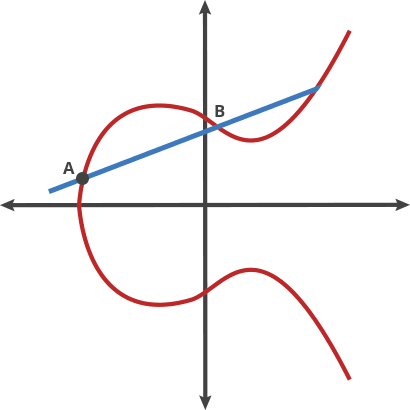A form of asymmetric cryptography which uses much smaller key-sizes than RSA

## Properties §

Specifically looking at Ed25519

The formula follows something like (which is symmetric about the x-axis)

Drawing a straight line through the curve will intersect no more than 3 points. A line between any two points will also intersect the curve at another place

Let the starting point on the curve point be point A. The “dot” function is kind of like a game of billiards.

In this game of billiards, you take a ball at point A, shoot it towards point B. When it hits the curve, the ball bounces either straight up (if it’s below the x-axis) or straight down (if it’s above the x-axis) to the other side of the curve. The point it lands on is C

If the value C is over some maximum value (usually a prime), we modulus it with the maximum to end with a valid number.

Illustrated example of A dot B = C:It turns out that if you “dot” an initial point with itself times to arrive at a final point, finding out when you only know the final point and the first point is hard.

is then the private key, point A dotted with itself times is the public key

## Key Exchange §

ECDHE stands for Elliptic Curve Diffie Hellman Ephemeral and is a key exchange mechanism based on elliptic curves

Curve25519 is a popular set of elliptic curve parameters and reference implementation by Daniel J. Bernstein in C Bindings and alternative implementations are also available.